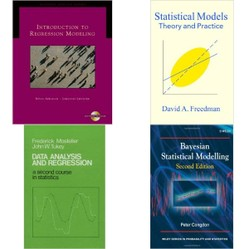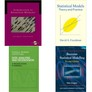# Linear Regression Books - Best Textbooks for Linear Regression

by cazort

## Reviews of textbooks in linear regression, oriented towards teachers, students, and career statisticians alike, with some mention of Bayesian regression.

Linear regression is a data-analysis technique and way of modelling data to assess the relationship between two variables. It is immensely useful and can be surprisingly fun!

Here I give personal reviews of books I worked with, mostly during my time studying for my master's degree in statistics from Yale University. I also have experience tutoring and teaching math, and working as a statistical consultant, and working in operations research.

I am sharing these reviews both for teachers and student, and for career statisticians or people using statistics in their work as well.

In addition to basic introductory texts at varying levels, I also include a more advanced book which covers Bayesian regression. This page is organized roughly in order of difficulty, with the more accessible books listed first. I have been highly selective of what books I include here, only reviewing books I find to be the best of the best in the subject of regression.

### A Word of Warning: Regression is Dangerous!

##### Linear regression is one of the most misused statistical techniques!

Linear regression is one of the most dangerous statistical techniques, in that it can be misused to draw false conclusions, because it is so convenient and so easy to work with, especially now, with the help of computers. When mis-used, regression can give you a neat formula describing the relationship between two variables, and can even provide you with statistical tests that tell you that the results are statistically significant. But these conclusions can be at best somewhat inaccurate and in some cases completely wrong because certain key assumptions underlying the model of linear regression are not met. My advice to avoid falling into this trap is as follows:

• Be a skeptic. Read books written by skeptics. - In order to use regression effectively, you need to be very cautious about knowing how and when to apply it. In many cases, regression is not an appropriate technique. Some statisticians talk about "skepticism" when teaching or working with regression. What others call skepticism, I call common sense. I think it is of key importance in working with regression to understand how and when it is appropriate, and to exercise restraint, and not use regression, when it is not the best technique to use.
• Consider thinking of regression as a data-analytic technique, not inference - The difference between inference and data analysis can be summed up as follows: data analysis describes the data itself, whereas inference tries to infer things about unknown quantities that go beyond what the data itself says. Regression is useful for both, but to be used for inference, it requires a statistical model which relies on many assumptions. When these assumptions are not satisfied, the usefulness of regression for inference is minimal.

I have hand-picked books that take this more skeptical, cautious, and rigorous approach. There are numerous widely-used books which I have chosen to omit from this list, because I feel they are careless in teaching people how to carry out regression without teaching them when, where, and why it is appropriate and when, where, and why it is not.

### Introduction to Regression Modeling

##### by Bovas Abraham and Johannes Ledolter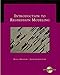Introduction to Regression Modeling (with CD-ROM) (Duxbury Applied)Using a data-driven approach, this book is an exciting blend of theory and interesting regression applications. Students learn the theory behind regression while actively applyi...View on Amazon

Abraham and Ledolter's Introduction to Regression Modeling is my single favorite introductory textbook for a course in regression; it also makes a good self-study text. Mathematically, it requires a certain comfort level with summation notation, and a working knowledge of linear algebra to get the most out of the book. A few optional sections on deeper topics may require a little more math. This book also requires some experience with data from the natural or social sciences, to get the most out of it.

Pros: Thorough, and easy-to-read. Goes unusually deep and covers a great deal of material, relative to how accessible it is. Provokes deep thought. Exhaustive coverage of topics makes this book a useful reference to own. The chapters on model selection and model checking are, in my opinion, an outstanding inclusion, as these are key factors in carrying out regression analysis that are often omitted from introductory textbooks. This book does an excellent job of integrating the practical and mathematical sides of the subject.

Cons: The price tag is quite high; this is an expensive book. It is worth it, though.

### Data Analysis and Regression: A Second Course in Statistics

##### by Frederick Mosteller and John W. Tukey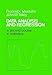Data Analysis and Regression: A Second Course in StatisticsBinding has slight discoloration. Rest of the text is flawless with crisp pages. Text is free of writing, marks, and highlighting. Hand inspected for quality and flaws.View on Amazon

This book is a classic text on linear regression which was written before the advent of modern computers. It has a very different way of presenting the subject, which I find emphasizing thinking more carefully about a dataset before starting to run computations on it.

Pros: I find the first two chapters of this book have a lot of timeless material in them. This book excels in the area of communicating the philosophy and approach to data analysis, teaching people how to think about the data and how to know when various techniques are important, and how to set them up. I feel these issues are often glossed over in modern textbooks, and for this reason I think this book is an excellent supplement to more modern, computer-oriented texts that move at a faster pace and provoke less philosophical reflection.

Cons: Because it written before modern computers, much of this book has become outdated or obsolete. Chapters 3-6 in particular are pretty obsolete, and are probably only worth reading for historical reasons.

### Regression Analysis: A Constructive Critique by Richard A. Berk

##### A deep and engaging book, but uses only constructed data sets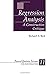Regression Analysis: A Constructive Critique (Advanced Quantitative Techniques in the Social Scie...Regression Analysis: A Constructive Critique identifies a wide variety of problems with regression analysis as it is commonly used and then provides a number of ways in which pr...View on Amazon

Linear regression and regression analysis in general are among the most often abused techniques in the field of statistics and data analysis. They can be used carelessly.

This book is specifically oriented towards the end of addressing these problems, and helping train statisticians in learning how to use regression properly, and how to avoid the major pitfalls of regression analysis.

Pros: Lively and captivating, this book is written about a subject that some people view as dry or boring, yet it's one of those books that you can't put down, like a thriller mystery novel. It covers the theory of regression but with an eye towards practical applications. The critical approach taken by this book makes it much easier to become a better statistician.

Cons: This book doesn't go into as much mathematical depth as a lot of intro texts on regression. It also does not cover. Lastly, there is a major lack of real data sets in the book--it consists almost exclusively of "toy" data sets, constructed by the author to illustrate a point. This may make the subject seem cleaner and more elegant than it is in practice. Lastly, this book barely mentions the Bayesian paradigm, which I think is a big omission.

Complemented by: Freedman's book provides a similar perspective, with more exposition of the mathematics.

### Statistical Models: Theory and Practice by David A. Freedman

##### Neat and clean, but limited in scope and too straightforward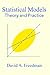Statistical Models: Theory and PracticeExplaining the things you need to know in order to read empirical papers in the social and health sciences, as well as techniques needed to build personal statistical models, th...View on Amazon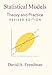Statistical Models: Theory and PracticeThis lively and engaging textbook explains the things you have to know in order to read empirical papers in the social and health sciences, as well as the techniques you need to...View on Amazon

Freedman's book Statistical Models is a very neat and clean introduction to the mathematical theory of regression, emphasizing both rigor and practical considerations. I find it would be excellent both as a textbook and self-study text, although it is a bit conventional and limited in scope. I think it is best supplemented it with other books.

Pros: Very clear, neat and clean presentation. Both accessible and rigorous. Linear algebra and mathematical notation is introduced gently and clearly. Exercises are very naturally graded in level of difficulty. The book progresses in a very natural way.

Cons: Limited in scope; covers only the basic techniques of linear regression. Does not mention Bayesian statistics, decision theory, quantile regression or other approaches to regression. Overly straightforward at times, and lacks philosophical depth. This book explains how to do linear regression well, and how the theory of it works, but it does little to explain how or why this theory was developed, or what to do in cases where it is not the most appropriate or useful technique.

### Bayesian Statistical Modelling by Congdon

##### An advanced book and reference, understating its prerequisites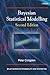Bayesian Statistical ModellingBayesian methods combine the evidence from the data at hand with previous quantitative knowledge to analyse practical problems in a wide range of areas. The calculations were pr...View on Amazon

Bayesian regression and Bayesian modeling and data-analytic techniques can be a good bit tougher to learn and master than vanilla, garden-variety linear regression. Appropriately, this book has a greater required background than any of the other books I've reviewed here, but to the right audience, it will be immensely useful. This is a book that will be most useful to advanced researchers and more experienced statisticians.

Pros: This book is very modern and up-to-date, integrating many newer techniques and touching on a lot of cutting-edge research. Rich citations to the primary literature are given, when relevant. I find the prose to be clearly written, and I think this book includes just the right amount of mathematical notation--enough to clarify, but not too much. It is also practical and data-oriented.

Cons: While I think this is an outstanding book overall, I think that the book's preface and back cover grossly understate the prerequisites necessary to understand the material. This book is very advanced, and I think that in order to be most useful to people, they need a solid background in the basics of Bayesian inference, thorough knowledge of basic regression, and some prior exposure to MCMC sampling.

### What exactly is "Bayesian Regression"?

##### A brief explanation of the field and approach of Bayesian statistics

Bayesian statistics is a branch of statistics, or a philosophy or approach within statistics, which involves interpretation of probability as a degree of belief. It is named for Thomas Bayes, pictured on the right, a statistician and theologian who did some early work which laid some of the foundation for the field that came to bear his name.

Bayesian linear regression is not well known, but there are some compelling reasons, both practical and philosophical, to use it rather than the mainstream linear regression techniques that were developed in the frequentist paradigm.

One key distinction of the Bayesian approach is that in the Bayesian paradigm, probabilities are interpreted as subjective degrees of belief. This allows both the incorporation of prior knowledge, which can lead to more accurate models if the priors are specified accurately. It also removes some of the problems with subjectivity in the frequentist paradigm: instead of model coefficients being accepted or rejected on the basis of statistical significance, Bayesian regression leads to a quantitative degree of certainty of what is known about various parameters.

### More Of My Math Textbook Reviews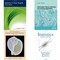Reviews of introductory textbooks in statistics, from more general-audience books to mathy books for advanced or graduate students.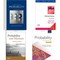Here I review textbooks in probability theory, from the introductory level through more advanced texts. I have chosen only books I consider to be the best of the best.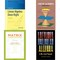Recommendations and reviews of textbooks for linear algebra at both undergraduate (college) and graduate levels.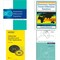Reviews of textbooks in differential equations or Diff-EQ, covering both ODE's and PDE's, and one general book on applied math.
Updated: 04/02/2015, cazort
2

Only logged-in users are allowed to comment. Login

#### You might also like

Probability Theory Books - Best Textbooks for Probability

Here I review textbooks in probability theory, from the introductory level th...

Linear Algebra Textbook Reviews

Recommendations and reviews of textbooks for linear algebra at both undergrad...

Disclosure: This page generates income for authors based on affiliate relationships with our partners, including Amazon, Google and others.